The SIMLIN Procedure

Multipliers for Higher Order Lags

The dynamic multiplier options require the system to have no lags of order greater than one. This limitation can be circumvented, since any system with lags greater than one can be rewritten as a system where no lag is greater than one by forming new endogenous variables that are single-period lags.

For example, suppose you have the third-order single equation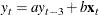This can be converted to a first-order three-equation system by introducing two additional endogenous variables, y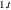and y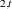, and computing corresponding first-order lagged variables for each endogenous variable: y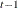, y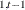, and y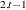. The higher order lag relations are then produced by adding identities to link the endogenous and identical lagged endogenous variables: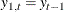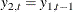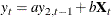This conversion using the SYSLIN and SIMLIN procedures requires three steps:

1. Add the extra endogenous and lagged endogenous variables to the input data set using a DATA step. Note that two copies of each lagged endogenous variable are needed for each lag reduced, one to serve as an endogenous variable and one to serve as a lagged endogenous variable in the reduced system.

2. Add IDENTITY statements to the PROC SYSLIN step to equate each added endogenous variable to its lagged endogenous variable copy.

3. In the PROC SIMLIN step, declare the added endogenous variables in the ENDOGENOUS statement and define the lag relations in the LAGGED statement.

See Example 25.2 for an illustration of how to convert an equation system with higher-order lags into a larger system with only first-order lags.